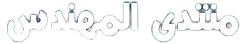# أرجوا منكم المساعدة اليوم ؟؟؟؟

أرجوا منكم المساعدة اليوم ؟؟؟؟

[FONT=Times New Roman]1
The surface area, A, of a hollow cone is given by the formula A = π r l
Find the surface area in square metres when r = 4m and l = 9m

2
In an electrical circuit the voltage V volts is given by V = IR
Find the voltage when I = 7.24 amperes and R = 12.57 ohms, correct to 4 significant figures

3
A formula used for calculating velocity v m/s is given by v = u + at
If u = 12.47 m/s, a = 5.46 m/s2 and t = 4.92 s find v correct to 2 decimal places

4
The are A m2 of a circle is given by A = πr2
Find the area correct to 2 decimal places given that r = 4.156 m

5
Power P watts in an electrical circuit may be expressed by the formula P = v2/R
Evaluate the power correct to 2 decimal places, given that V = 24.62 volts and R = 45.21 ohms

6
The volume V m3 of a right circular cone is given by the formula V = 1/3 πr2h
Given r = 5.637 m and h = 16.41 m, find the volume in standard form correct to 3 significant figures

[/font]

أرجوا منكم المساعدة